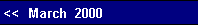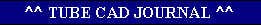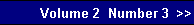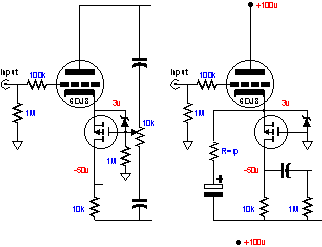The formula is as follows:   PSRR = 20Log(Ra / (rp + Rk(mu + 1)In this example:    -6 dB = 20Log(10k / (3.3k + 205(33+1)))Now, -6 dB means that the voltage division is equal to one half. In other words, the triode and cathode resistor combination equals 10k. And since a bipolar power supply should exhibit equal, but anti-phase noise on each leg, the power supply noise at the plate should equal zero. This assumes that the triode's grid is tied to the negative rail. If the grid were referenced to ground through a coupling capacitor, the negative power supply rail noise would be greatly amplified and would overwhelm the positive rail noise. So, here is the beauty of using the inverted cascode first: it really does not reject power supply noise. Thus, what noise appears at the negative rail will also appear at the top of the Cascode's load resistor.   Well, sort of. The rosy picture darkens when we realize that the input triode at the base of the Inverted Cascode has rp and that this resistance to voltage movement at its plate will give rise to a noise current that will be relayed to the load resistor. Since the load resistor is about threefold greater in value than the rp of the
 tube, we can expect a threefold increase in noise at the top of the load resistor. It does not matter that the negative rail noise is in anti-phase to this noise current, as the noise the bottom triode sees is referenced to the negative rail and not ground.  (On the other hand, if we let the load resistor equal the rp of the tube, the power supply noise will fall out of the output when referenced to ground, which would be useful if the second stage were referenced to ground.)Alternatively, one of the two circuit variations above could be used to cancel the positive power supply noise from the load resistor.pg. 6